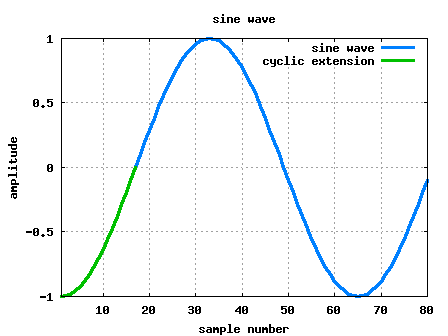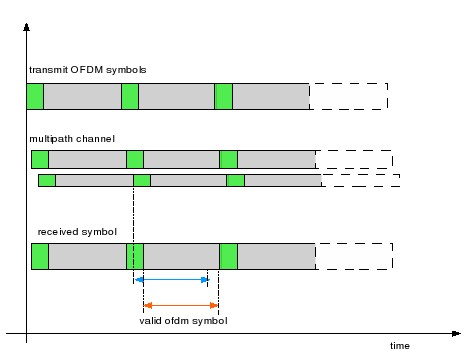- DSP log - http://www.dsplog.com -

Cylcic prefix in Orthogonal Frequency Division Multiplexing

Posted By Krishna Sankar On February 17, 2008 @ 3:19 pm In OFDM | 55 Comments

In a previous post (here ), we discussed in brief, Orthogonal Frequency Division Multiplexing (OFDM) transmission. Let us know probe bit more into the motivation of cyclic prefix (aka guard interval) associated with each OFDM symbol.

What is cyclic prefix?
Let us consider one subcarrier (subcarrier +1 specified in IEEE 802.11a specification ) alone. In the figure shown below, the blue line corresponds to the original sinusoidal where one cycle of the sinusoidal is of duration 64 samples ( with 20MHz sampling), corresponding to subcarrier of frequency 312.5kHz.Figure: Sinuosoidal with cyclic prefix

To add the cyclic prefix, 16 samples () from the end of the sinusoidal are appended to the beginning of the sinusoidal (shown in green colour). As can be seen, appending of cyclic prefix does not cause any discontinuities and we still have the original sinusoidal of frequency 312.5kHz.

Further, after adding cyclic prefix, as the sinusoidal is of duration , we now have a bigger window for choosing one period of the sinuosidal. Ofcourse, depending on which set of is chosen, the phase needs to be corrected, but that will be a trivial operation in a typical implementation.

Effect of passing a sinusoidal through a multipath channel
Let us consider a simple multipath channel of the form
.
Let the transmit symbol be a single sinusoidal .
.

As can be seen from the above equation, after passing through the multipath channel, the received signal is the original sinusoidal albeit with modifications in amplitude and phase.

Use of cyclic prefix in multipath channel
Cyclic prefix acts as a buffer region where delayed information from the previous symbols can get stored. The receiver has to exclude samples from the cyclic prefix which got corrupted by the previous symbol when choosing the samples for an OFDM symbol. Further, from the previous section, we learned that a sinusoidal added with a delayed version of the same sinusoidal does not affect the frequency of the sinusoidal (it only affects the amplitude and phase).Figure: OFDM symbol with multipath

Given so, for demodulating the received symbol, the receiver can chose samples from a region which is not affected by the previous symbol. As shown in the figure above, the samples can be chosen from the blue-arrow region OR the orange-arrow region OR somewhere inbetween.Note:
When the pertinent samples of an OFDM symbol is taken (as explained above), the orthogonality aspect in OFDM is not affected by the multipath channel i.e. eventhough the individual subcarriers undergo phase and amplitude change, as the frequency is not affected, there is no interference between the subcarriers.

Cons of cyclic prefix
Ofcourse, the flipside of adding cyclic prefix is the loss in data rate as we are conveying redundant information.

Choosing the cyclic prefix duration
Given that transmission of cyclic prefix reduces the data rate, the system designers will want to minimize the cyclic prefix duration. Typically, cyclic prefix duration is determined by the expected duration of the multipath channel in the operating environment. For example, for the indoor wireless multipath channel, the typically expected multipath channel is of around duration, hence determining the cyclic prefix chosen per the IEEE 802.11a specification .

Summary
1. Passing a sinsuodial through a multipath channel does not affect the frequency of the sinusoidal.

2. As the multipath channel does not change the frequency of the sinusoidal, multipath channel does not affect orthogonality of the subcarriers (only if the receiver chooses samples which correspond only to the delay-added versions of the samples of the current symbol).

3. Transmission of cyclic prefix reduces the data rate, hence the cyclic prefix duration should not be much more than the duration of the maximum expected multipath channel.

Hope this helps.
Krishna